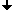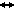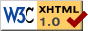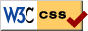Nonlinear Dynamics at the Free University Berlin

Winter 2012/2013

Seminar Advanced Topics in Nonlinear Dynamics

PD Dr. P. Gurevich, Dr. Stefan Liebscher

Program

 Oct 18, 2012 Bernhard Brehm(Free University Berlin) Complexified transit estimates for Bianchi 8 and 9 cosmologies in the asymptotic Bianchi II regime It is a well-established heuristic that most orbits for Bianchi 8 and Bianchi 9 cosmologies are at late times well approximated by a sequence of Bianchi 2 orbits. I will use this heuristic to construct an approximate Poincare-map $\Phi_0$ and will show that this map approximates the actual return-map in the C^0-norm. Since the Bianchi ODE is polynomial, we can complexify it and will prove that (in a reasonable domain) the same estimates hold, thus yielding $C^\infty$-estimates via Cauchy's integral formula. I will present (and roughly sketch the proof of) two corollaries of these estimates, which can be summarised by the following: 1. Stable foliation. For almost every base-point, there is an analytic (in the interior) codimension 1 stable manifold attached. 2. Positive measure. The union of these attached stable manifolds has positive measure. Oct 25, 2012 Klaus Ecker(Free University Berlin) Entropy and Harnack type formulas for evolving domains We present a notion of entropy for domains in Eulcidean space which modifies Perelman's entropy introduced for closed Riemannian manifolds. We'll discuss basic properties of this quantity and explain how it is related to control of local volume ratios for the domains under consideration and how it may prevent local volume collapse for families of evolving domains. If time permits we will also show a natural connection between the entropy and Harnack inequalities for the backward heat equation. Nov 1, 2012 Alan Rendall / Juliette Hell(Albert-Einstein-Institute Golm / Free University Berlin) Relaxation Oscillation in the MAP-kinase Cascade We introduce the MAP-kinase cascade, a pattern of chemical reactions which is an important element of many signaling pathways in cells. This process may be modelled in different ways. A common feature of models/numerical simulations/experiments is the existence of periodic orbits due to relaxation oscillation. We will present a rigorous proof of this phenomenon for a model with feedback control, due to Gedeon and Sontag; express some critique of their model and results; and finally discuss the model we propose to study. Nov 8, 2012 Juliette Hell (Free University Berlin) Diffusion induced blow up I will report on an example by Ninomiya et al. where an ode system with a global attractor combined with a diffusion term gives surprisingly rise to blow up solutions. This phenomenon reminds of Turing instability where the diffusion destabilizes a stable ode equilibrium. Nov 15, 2012 Hannes Stuke(Free University Berlin) Blow-up in complex time I will discuss the blow-up behaviour of the one-dimensional heat equation with quadratic nonlinearity in complex time. The first talk will give an introduction to the problems of: 1. Existence and uniqueness of solutions 2. Global behaviour 3. Analytic continuation beyond the blow-up time. Nov 22, 2012 Tobias Marxen(Free University Berlin) Ricci flow of noncompact warped product manifolds We define the Ricci flow on R^n and show that certain warped product solutions of Ricci flow are equivalent to solutions of a system of PDE�s. Next we explain how one can get estimates for geometric quantities by means of a maximum principle. We sketch what collapsing of such a warped product solution means. Finally we indicate how one can obtain Gaussian estimates for Ricci flow by considering the example of the heat equation on R^n. Nov 29, 2012 Vladimir Popov(Peoples' Friendship University of Russia Elliptic functional differential equations with degeneration We consider elliptic differential-difference equations with several nonnegative difference operators. The interest in such operators is due to their fundamentally new properties compared with even strongly elliptic differential-difference operators as well as due to applications of the obtained results to certain nonlocal problems arising in the plasma theory. We obtain a priori estimates of solutions. In addition, using these estimates, we can show that the considered operator is sectorial, construct its Friedrichs extension, and prove a theorem on the smoothness of solutions. Nov 29, 2012 Markus Mittnenzweig(Free University Berlin) Optimal transport and gradient flows in the Wasserstein space I will give an introduction to the theory of Optimal Transport. This notion defines a natural metric - the Wasserstein distance - in the space of probability measures. I will show that certain types of PDEs can be viewed as gradient flows with respect to that metric and how this interpretation can be used to establish estimates for convergence rates in these equations. Dec 6, 2012 Sergey Tikhomirov(Free University Berlin) Shadowing in actions of finitely generated groups We consider one of the possible generalizations of the notion of shadowing property to actions of nonabelian groups. We consider how the classical shadowing lemma can be generalized for this case. An important example will be Baumslag-Solitar group. Dec 13, 2012 Johannes Buchner(Free University Berlin) Dynamics of Bianchi Cosmologies, the BKL Conjecture and the Tumbling Universe The BKL-Conjecture states that the approach to the initial singularity is vacuum-dominated, local and oscillatory. The highly symmetric Bianchi cosmologies play an important role in this BKL-picture, as they are believed to capture the essential dynamics of more general solutions. A detailed study of Takens Linearization Theorem and the Non-Resonance-Conditions lead us to a new result in Bianchi class A: We are able to show, for the first time, that for admissible periodic heteroclinic chains in Bianchi IX there exisist C1- stable - manifolds of orbits that follow these chains towards the big bang. We also study Bianchi models of class B, where no rigorous results exist to date. We find an example for a periodic heteroclinic chain that allows Takens Linearization at all Base points and give some arguments why it qualifies as a candidate for proving the first rigorous convergence theorem in class B. We conclude with an outlook on future research on the chaotic dynamics of the Einstein Equations towards the big bang - in order to shed a little more light on our "tumbling universe" at birth. Jan 10, 2013 Oleh Omelchenko(WIAS) Thermodynamic limit approach for bifurcation analysis of chimera states Chimera states are coherence-incoherence patterns observed in homogeneous discrete oscillatory media with non-local coupling. Despite their nontrivial dynamical nature, such patterns can be effectively analyzed in terms of the thermodynamic limit formalism. In particular, using statistical physics concept of local mean field and the Ott-Antonsen invariant manifold reduction, one can explain typical bifurcation scenarios leading to the appearance of chimera states and provide their reasonable classification. Jan 17, 2013 Bernhard Brehm(Free University Berlin) Proof of complexified transit estimates in Bianchi 8 and 9 cosmologies In Bianchi 8 and 9 cosmologies, estimates for the transit near the Kasner circle of equilibria are essential for questions regarding long-time dynamics. In the fall 2012, I presented new estimates on the transit in a complexified version of the Bianchi differential equations (thus allowing to obtain estimates on derivatives by Cauchy integrals). This talk will focus on the technical details of the proof of these estimates, using a perturbative ansatz, elementary but lengthy estimates on various integral operators and a variant of Schauder's fixed point theorem. Jan 31, 2013 Jia-Yuan Dai(Free University Berlin) Spiral patterns: model derivation and existence of rotating spirals In this talk, I will briefly explain the phenomena such as the Belousov-Zhabotinsky reaction to show how spiral patterns arise and why they are important and interesting. Then we may ask: What are the corresponding mathematical models for spiral patterns? The most intuitive way is perhaps the reaction-diffusion systems. I will show how to derive the systems from the special Euclidean symmetry SE(2). Another approach is the kinematic model. It regards a spiral as a curvature flow along the normal direction of a given planar curve. At last, I will focus on the kinematic model and show how to prove the existence of rotating spirals. Feb 7, 2013 Markus Baer(Physikalisch-Technische Bundesanstalt, Berlin) A poroelastic model for mechanochemical waves and pattern formation in Physarum polycephalum Many processes in living cells are controlled by biochemical substances regulating active stresses. The cytoplasm is an active material with both viscoelastic and liquid properties. First, we incorporate the active stress into a two-phase model of the cytoplasm which accounts for the spatiotemporal dynamics of the cytoskeleton and the cytosol. The cytoskeleton is described as a solid matrix that together with the cytosol as interstitial fluid constitutes a poroelastic material. We find different forms of mechanochemical waves including traveling, standing and rotating waves by employing linear stability analysis and numerical simulations in one and two spatial dimensions. In a second step, we expand the chemo-mechanical model in order to model the manifold contraction patterns observed experimentally in protoplasmic droplets of Physarum polycephalum. To achieve this, we combine a biophysically realistic model of a calcium oscillator with the poroelastic model derived in the first part of the talk and assume that the active tension is regulated by calcium. With the help of two-dimensional simulations the model is shown to reproduce the contraction patterns observed in protoplasmic droplets as well as a number of other traveling and standing wave patterns. Joint work with Markus Radszuweit (PTB, TU Berlin), S. Alonso (PTB), H. Engel (TU Berlin). Feb 14, 2013 Konstantin Bubolz, Matthias Bosewitz, Alexander Sikorski, Anna Karnaukhova, Phillipo Lapicy (Free University Berlin) Ongoing research

Time and Place

Talks usually take place on Thursday at 2:15 p.m.
at the Free University Berlin
Room 130, Arnimallee 3 (rear building), 14195 Berlin.

Guests are always welcome!

ArchiveLast change: Feb. 21, 2013
 This page strictly conforms to the XHTML1.0 standard and uses style sheets.